# Finding the coordinate of a point by Law of Cosines/Sines

Alison A.

## Homework Statement

A model for the suspension of a vehicle is shown where the spring has stiffness k = 178 N.mm and an unstretched length of 347 mm.

Here is the picture: http://i.imgur.com/1dTVs12.jpg

Part a asked to determine the value of P and the force supported by member AB so that the suspension was in equilibrium, and I got that right.

However part b asks "If P = 0, determine the x and y coordinates of point A, namely xA and yA."

Law of sines
Law of cosines
Basic trig

## The Attempt at a Solution

I used the law of cosines to find all the angles
A = 15.737 degrees
B = 138.128 degrees
C = 26.135 degrees

I don't know where to go from here to find the coordinates, I feel like this is probably really easy but I'm over thinking it.

Thank you so much for your help!

Staff Emeritus
Homework Helper

## Homework Statement

A model for the suspension of a vehicle is shown where the spring has stiffness k = 178 N.mm and an unstretched length of 347 mm.

Here is the picture: http://i.imgur.com/1dTVs12.jpg

Part a asked to determine the value of P and the force supported by member AB so that the suspension was in equilibrium, and I got that right.

However part b asks "If P = 0, determine the x and y coordinates of point A, namely xA and yA."

Law of sines
Law of cosines
Basic trig

## The Attempt at a Solution

I used the law of cosines to find all the angles
A = 15.737 degrees
B = 138.128 degrees
C = 26.135 degrees

I don't know where to go from here to find the coordinates, I feel like this is probably really easy but I'm over thinking it.

Thank you so much for your help!
You've calculated three angles, the distances AB and BC are shown in the diagram, and points B and C are fixed to the vehicle.

If nothing else, you could make a sketch and find the third distance.

In case you've forgotten how to apply the Law of Sines, here is a picture: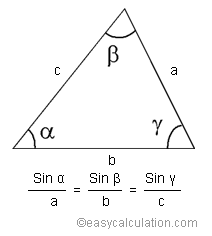Homework Helper
Are you considering point B to be your origin?
In that case, you can draw a right triangle with hypotenuse of length 229mm, and determine the angles (B-90), 90, (180-B). From there, the calculations are 229mm(sine ) and 229mm(cosine). Then be sure to orient your vectors in the proper direction.

Alison A.
You've calculated three angles, the distances AB and BC are shown in the diagram, and points B and C are fixed to the vehicle.

If nothing else, you could make a sketch and find the third distance.

In case you've forgotten how to apply the Law of Sines, here is a picture: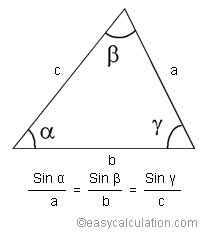I did that, and I got the same lengths, I don't need to find the lengths I need to find where the coordinates are : /

Alison A.
Are you considering point B to be your origin?
In that case, you can draw a right triangle with hypotenuse of length 229mm, and determine the angles (B-90), 90, (180-B). From there, the calculations are 229mm(sine ) and 229mm(cosine). Then be sure to orient your vectors in the proper direction.

B is my origin because it is fixed in place and when the forces aren't exerted on AB or the spring the hinge swings open with B fixed in place. I don't get what you mean when you say
(B-90), 90, (180-B).

Staff Emeritus
Homework Helper
I did that, and I got the same lengths, I don't need to find the lengths I need to find where the coordinates are : /
You use the lengths of the sides and a little geometry to determine the coordinates. The (x,y) coordinate of point A is not just going to appear. You definitely should make a sketch of your triangle before working out the location of point A.

Homework Helper
B is my origin because it is fixed in place and when the forces aren't exerted on AB or the spring the hinge swings open with B fixed in place. I don't get what you mean when you say

You have the angles:
B = 138.128 degrees.
If you draw the x-axis, you will see that the right triangle formed with B, A, and the axis will have one angle measuring (138.128-90)degrees.
The hypotenuse is equal to 229mm according to your drawing.
Then use sine = opposite / hypotenuse to solve for the y component (negative) and cosine = adjacent/hypotenuse to solve for the x component.

Alison A.
Sin(138.128)/347=Sin(26.135)/c
c = -2869.62

Sin(138.128)/347=Sin(15.737)/a
a = 98.8653

Alison A.
You use the lengths of the sides and a little geometry to determine the coordinates. The (x,y) coordinate of point A is not just going to appear. You definitely should make a sketch of your triangle before working out the location of point A.

Sin(138.128)/347=Sin(26.135)/c
c = -2869.62

Sin(138.128)/347=Sin(15.737)/a
a = 98.8653

Homework Helper
That number for c doesn't seem reasonable, and doesn't follow from the math. You are still using the law of sines to tell you what you already know...only now you are doing the calculations incorrectly. (Check degrees and not radians).

Alison A.
That number for c doesn't seem reasonable, and doesn't follow from the math. You are still using the law of sines to tell you what you already know...only now you are doing the calculations incorrectly. (Check degrees and not radians).

a = 141.001
b = 228.998

Which is exactly the information the problem already provides.

Homework Helper
Draw a right triangle.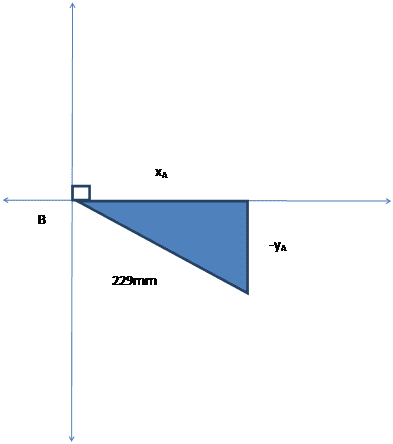Homework Helper
What should the measure of the angle at B be?
Call that angle m.
sin(m) = opp/hyp
Does that make more sense?

Alison A.
Draw a right triangle.
View attachment 89094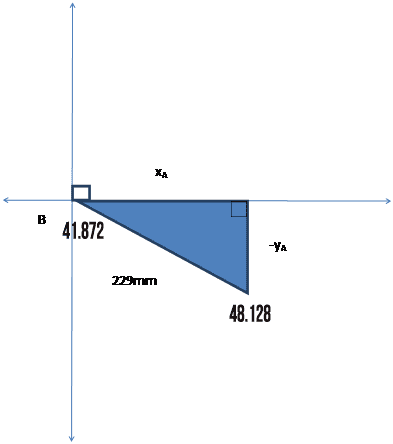Homework Helper
Why would 48.128 be down there? Wasn't that part of angle B? 90+48.128 = 138.128.

Alison A.
Why would 48.128 be down there? Wasn't that part of angle B? 90+48.128 = 138.128.

I don't know, I was trying to do what you mentioned before about (B-90), 90, (180-B)

Homework Helper
You shouldn't just blindly put the angles in. Use what you know. If the angle at B was 138.128, and you just keep the part that falls below the x-axis, how much is left?
That part is still at point B in the diagram.

Alison A.
You shouldn't just blindly put the angles in. Use what you know. If the angle at B was 138.128, and you just keep the part that falls below the x-axis, how much is left?
That part is still at point B in the diagram.

I didn't think I put them in randomly... Did we cut the triangle in half somewhere? I'm still not sure how we got this right triangle.

EDIT: So the part that is still at point B would be 138.128-90 right? Do I just have the numbers switched?

Alison A.
I didn't think I put them in randomly... Did we cut the triangle in half somewhere? I'm still not sure how we got this right triangle.

EDIT: So the part that is still at point B would be 138.128-90 right? Do I just have the numbers switched?

This homework is due in an hour and is 10 out of the 30 pointsplease help

Homework Helper
Your edit is right. We didn't move anything, just ignored the first 90 degrees of the angle to make a right triangle below.

Alison A.
Alright, I got the problem wrong too many times so now I have new values. B is now 104.99. I tried taking sin(90)/323 = sin(14.99)/-y, making y -83.544 but that wasn't right...

Alison A.
Alright, I got the problem wrong too many times so now I have new values. B is now 104.99. I tried taking sin(90)/323 = sin(14.99)/-y, making y -83.544 but that wasn't right...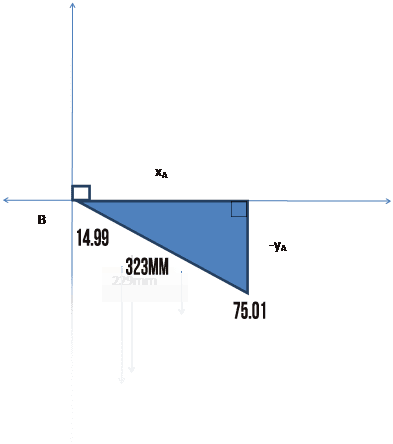Staff Emeritus
Homework Helper
It's not clear what you are doing here.

Arm AB has a fixed length of 229 mm, which doesn't change as the tire moves up and down.

The distance BC is also fixed at 141 mm.

The distance AC changes as the spring is stretched or compressed by the movement of the tire up and down.

Alison A.
It's not clear what you are doing here.

Arm AB has a fixed length of 229 mm, which doesn't change as the tire moves up and down.

The distance BC is also fixed at 141 mm.

The distance AC changes as the spring is stretched or compressed by the movement of the tire up and down.

I don't know... I'm so confused... I can find the angle of B by law of cosines
(323)^2=(169)^2+(235)^2-2(169)(235)cosB
B = 104.99 degrees

From here, I really have no idea.

Alison A.
Here is the new problem statement if you are confused about the new numbers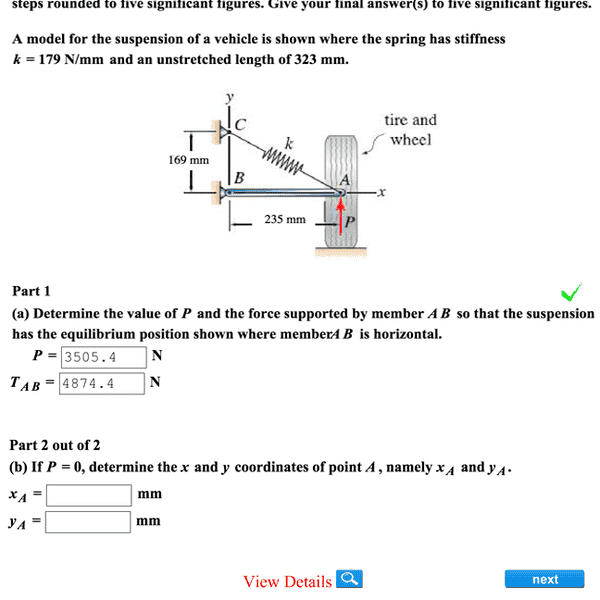Staff Emeritus
Homework Helper
This is confusing. If you have a new problem, please post it in a new thread.

Alison A.
This is confusing. If you have a new problem, please post it in a new thread.
It's not a new question... I answered it too many times so they changed the values. I don't need help with Part A I have already done it as you can see by the check mark.

Homework Helper
You had the right method but the wrong hypotenuse. In the diagram, the hypotenuse of this right triangle is the fixed rod (235mm) not the spring.

•Alison A.
Alison A.
Thank you so much, I got the right answer. That made a lot more sense that it was the rod and not the spring. Thank you both for not giving up on me!•RUber